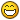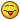# LookAt / Rotate towards a point using only x and y coordinates

## Recommended Posts

Hi,

Is there a built in way to "lookAt" a point in 3D space, using only 2 coordinates( x and y ).
I found a lookAt function from this forum, but that used 3 coordinates which screws up the camera and player orientation.
Id like to keep my player's z rotation always at 0.

##### Share on other sites

Hi @rainerpl

We can accomplish this by making a function,

we'll simply borrow the 2d rotation parts of the lookAt function, mainly

``````var dv = targetPoint.subtract(this.position);
var yaw = -Math.atan2(dv.z, dv.x) - Math.PI / 2;``````

now we insert it in a function..

``````function lookAt(tM, lAt) {
/*
* tM = mesh to rotate.
* lAt = vector3(xyz) of target position to look at
*/

lAt = lAt.subtract(tM.position);
tM.rotation.y = -Math.atan2(lAt.z, lAt.x) - Math.PI/2;
}``````

and finally we use it like this;

``````//mesh = Mesh we want to rotate
//target = Target point to make the mesh look at.

lookAt(mesh, target);``````

PG; //Click on the ground to rotate the box##### Share on other sites

Hi rainerpl and welcome to the forum!

##### Share on other sites

• 4 weeks later...
On 2016/10/12 at 5:57 PM, iiceman said:

Hi rainerpl and welcome to the forum!

I have a question.

How to change its rotation in 3D space, not only change rotation.y.##### Share on other sites

@HenryPeng I am afraid I don't have a good answer. I remember I tried to achieve the same when I played with this here: http://p215008.mittwaldserver.info/space/ if you click at an asteroid the ship is supposed to smoothly align with the target and then shoot. But if I remember right it doesn't always work, so I guess there is a flaw in my way of doing it. I also remember I have tried a lot of things but it was never perfect. Maybe somebody else has a good idea how to achieve the in a way that's more...bulletproof. :-/

I think those where the important functions:

``````/**
* @param rotatingObject
* @param pointToRotateTo
* @returns {boolean}
*/
function facePoint(rotatingObject, pointToRotateTo) {

// a directional vector from one object to the other one
var direction = pointToRotateTo.subtract(rotatingObject.position);
var distanceToTargetPoint = direction.length();

if(distanceToTargetPoint > 0.5) {

var axisVectorY = new BABYLON.Vector3(0, 0, 1);
var directionAxisForY = 'x';
var deltaY = calculateRotationDeltaForAxis(rotatingObject, 'y', axisVectorY, direction, directionAxisForY);

var axisVectorZ = new BABYLON.Vector3(0, 1, 0);
var directionAxisForZ = 'z';
var deltaZ = calculateRotationDeltaForAxis(rotatingObject, 'z', axisVectorZ, direction, directionAxisForZ);

var turnAroundYAxisDone = applyRotationForAxis(rotatingObject, 'y', deltaY);
var turnAroundZAxisDone = applyRotationForAxis(rotatingObject, 'z', deltaZ);

return (turnAroundYAxisDone && turnAroundZAxisDone) ? true : false;

}
}

function faceTarget(rotatingObject, target){
return facePoint(rotatingObject, target.position);
}

function calculateRotationDeltaForAxis(rotatingObject, axis, axisVector, direction, directionAxis){
var axisVectorNormalized = axisVector.normalize();
var directionVectorNormalized = direction.normalize();

// calculate the angel for the new direction
var angle = Math.acos(BABYLON.Vector3.Dot(axisVectorNormalized, directionVectorNormalized));

if (directionAxis == 'x') {
// decide it the angle has to be positive or negative
if (direction[directionAxis] < 0) angle *= -1;
// compensate initial rotation of imported spaceship mesh
angle += Math.PI / 2;
} else {
angle -= Math.PI / 2;
}

// calculate both angles in degrees
var playerRotationOnAxis = rotatingObject.rotation[axis];
// calculate and return the delta
return playerRotationOnAxis - angle;
}

function applyRotationForAxis(rotatingObject, axis, delta){
var pi = Math.PI;

// check what direction to turn to take the shortest turn
if (delta > pi) {
delta -= pi*2;
} else if (delta < -pi) {
delta += pi*2;
}

var absDelta = Math.abs(delta);
// rotate until the difference between the object angle and the target angle is no more than 3 degrees
if (absDelta > pi/360) {

var rotationSpeed = Math.max(0.2, Math.min(absDelta*absDelta, 1));

if (delta > 0) {
rotatingObject.rotation[axis] -= rotationSpeed * 0.1;
if (rotatingObject.rotation[axis] < -pi) {
rotatingObject.rotation[axis] = pi;
}
}
if (delta < 0) {
rotatingObject.rotation[axis] += rotationSpeed * 0.1;
if (rotatingObject.rotation[axis] > pi) {
rotatingObject.rotation[axis] = -pi;
}
}

// turn not done yet
return false;

} else {

// turn done
return true;

}
}``````

##### Share on other sites

17 minutes ago, iiceman said:
```
```    /**
* direction vector x,y,z,
* @param x
* @param y
* @param z
* @param defaultVec default direction(just you set)
*/
function getFaceRotation(x, y, z, defaultVec) {
defaultVec = defaultVec || new BABYLON.Vector3(0,0,1); //forward
var v1 = new BABYLON.Vector3(x,y,z);
v1.normalize();

var v2 = defaultVec;
var axis = BABYLON.Vector3.Cross(v2, v1);
axis.normalize();
var angle = Math.acos(BABYLON.Vector3.Dot(v1, v2));

var rotation = BABYLON.Quaternion.RotationAxis(axis, angle).toEulerAngles();
return rotation
}``````

I think it mybe a way##### Share on other sites

Well, here is a playground with my version... http://www.babylonjs-playground.com/#1SP0QL#0 ... it demonstrates that mine is no good... some hit pretty good, some almost..and some are pretty far off. I am nor exactly sure how to use yours, maybe you can make a playground, too?

## Join the conversation

You can post now and register later. If you have an account, sign in now to post with your account.
Note: Your post will require moderator approval before it will be visible.×   Pasted as rich text.   Paste as plain text instead

Only 75 emoji are allowed.